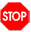> {-# LANGUAGE GADTs #-}

# Module 05: The Arith language: pretty-printing

• Write your team names here:

• You may again choose whoever you want to start as the driver. Write your choice here:

## Arith syntax and semantics

The Arith language is represented by the following abstract syntax:

> data Arith1 where
>   Lit1 :: Integer -> Arith1
>   Add  :: Arith1 -> Arith1 -> Arith1
>   Sub  :: Arith1 -> Arith1 -> Arith1
>   Mul  :: Arith1 -> Arith1 -> Arith1
>   deriving (Show)
>
> arithExample :: Arith1
> arithExample = Add (Mul (Lit1 4) (Lit1 5)) (Lit1 2)

(We are using the name Arith1 to avoid a name clash, since later we will use a more refined version called Arith.) The semantics of an Arith expression is an integer: Lit1 values represent themselves, Add represents addition, Sub subtraction, and Mul multiplication. For example, arithExample evaluates to $$(4 \times 5) + 2 = 22$$.

• Write an interpreter called interpArith1 for Arith1 expressions.

As concrete syntax for Arith, we use standard mathematical notation and standard conventions about operator precedence. For example, "4*5+2" is concrete syntax for arithExample. Notice that it does not represent Mul (Lit1 4) (Add (Lit1 5) (Lit1 2)) (which evaluates to 28), since by convention multiplication has higher precedence than addition. If we wanted the latter Arith1 value, we would have to write "4*(5+2)".

• Write a pretty-printer prettyArith1 which turns Arith abstract syntax into valid concrete syntax. At this point, you should try to make your pretty-printer as simple as possible rather than try to produce the best output possible. (Hint: something like "((4*5)+2)" is just as valid concrete syntax as "4*5+2", even though it has unnecessary parentheses.)

• How might you go about altering your pretty printer to omit needless parentheses? Write down some ideas here.

## Class discussion: precedence## A better pretty-printer

• ROTATE ROLES and write the name of the new driver here:
> data Op where
>   Plus  :: Op
>   Minus :: Op
>   Times :: Op
>   deriving (Show, Eq)
>
> data Arith where
>   Lit :: Integer -> Arith
>   Bin :: Op -> Arith -> Arith -> Arith
>   deriving (Show)
>
> data Associativity where
>   L :: Associativity
>   R :: Associativity
>   deriving (Show, Eq)
>
> type Precedence = Int
• First, write an interpreter interpArith for Arith expressions.

• Write functions assoc :: Op -> Associativity and prec :: Op -> Precedence to return the associativity and precedence of each operator. Addition, multiplication, and subtraction are all left-associative by convention. Addition and subtraction should have the same precedence level, with multiplication at a higher level (typically larger numbers represent higher precedence).

• Now write a function prettyPrec :: Precedence -> Associativity -> Arith -> String. Given the precedence level of the parent operator and whether the current expression is a left or right child, it should print out a properly parenthesized version of the expression, with parentheses surrounding the entire expression only if they are needed.

• Finally, write a function prettyArith :: Arith -> String which works by calling prettyPrec with appropriate starting arguments.